Cats2D Multiphysics > Research Topics > A difficult issue for elliptic mesh generation

A difficult issue for elliptic mesh generation

I have collated some entries from my developments page into this article that evaluates different approaches of handling irregular points in structured meshes computed by elliptic mesh generation.

A quick primer on elliptic mesh generation (EMG)

Cats2D uses elliptic mesh generation on a structured mesh of quadrilateral regions. The user is responsible for decomposing the domain into these regions. This aspect of Cats2D is one of its greatest weaknesses. It requires significant user effort. The best arrangement of regions is not necessarily unique or obvious, and domains with convoluted boundaries or sharp corners can be problematic.

Here is one way to decompose a circle, with a diagram showing the logical arrangement of regions in the computational space. Compass directions must be assigned to each region to define its orientation with respect to the other regions.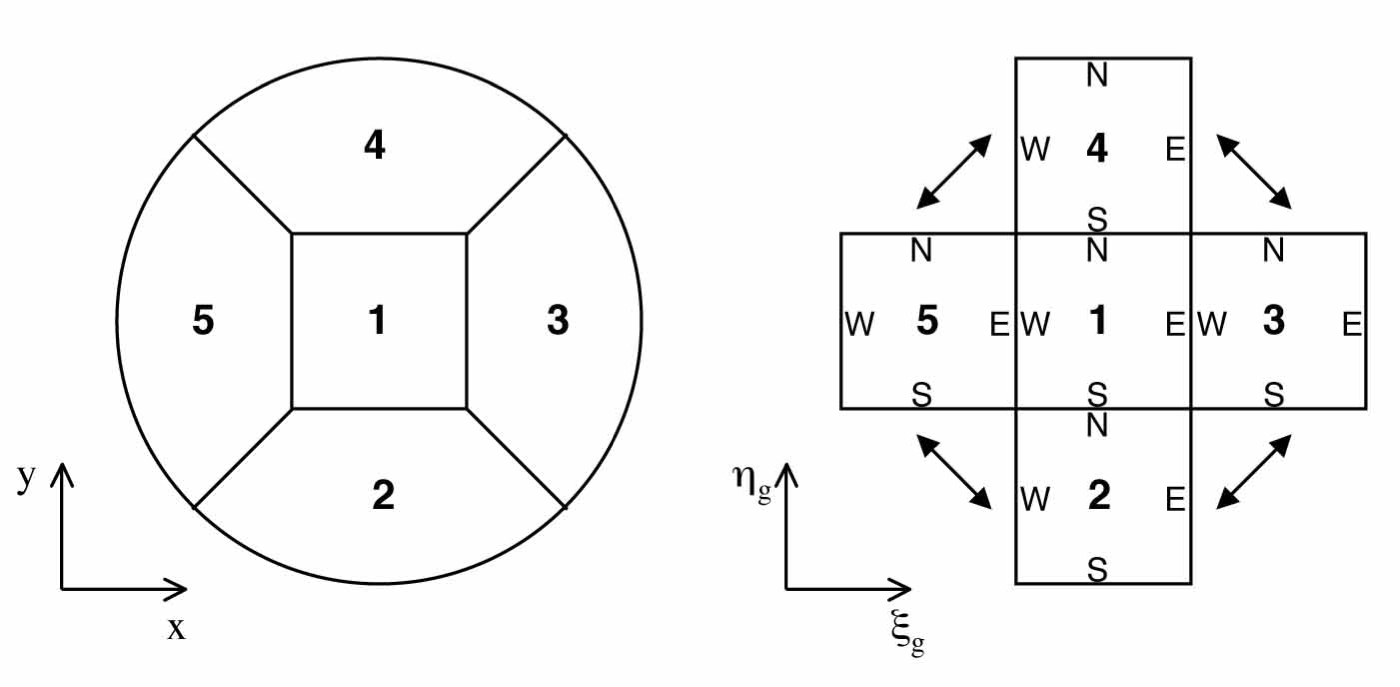One of these regions is shown below on the left, divided into a 6x6 array of finite elements. The individual elements are mapped from the physical space (X,Y) to a reference space (Xi,Eta) that we call the parent element on which the equations are discretized. The region as a whole is mapped to a computational domain of parent elements, shown on the right.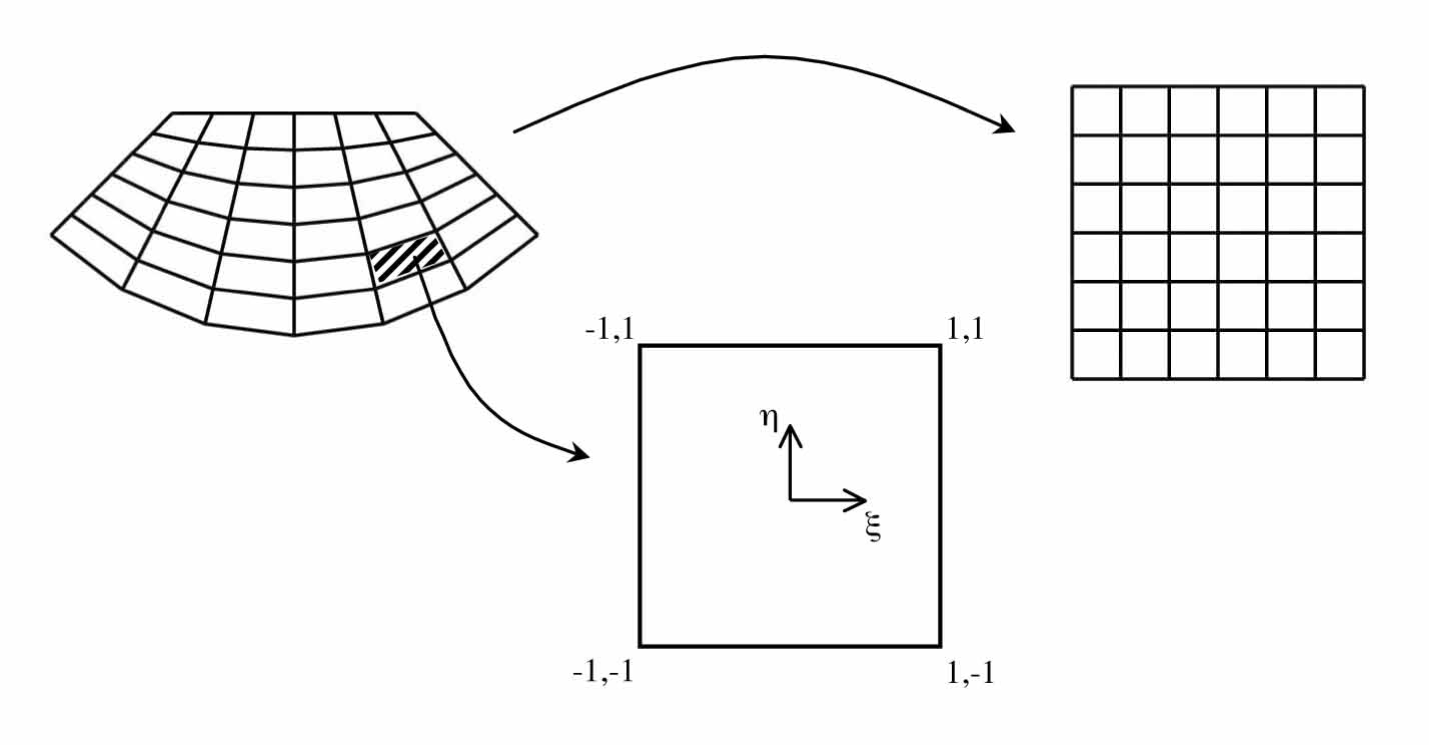Commonly the shape and size of the elements in the physical space is determined by an algebraic interpolation from the boundary discretization, such as transfinite interpolation. But for deforming boundary problems it can be desirable to apply a mapping from the computational space Xi,Eta to the physical space X,Y as a means to specify the deformation of elements, which makes it easy to couple mesh deformation with physics.

This can be done by defining the mesh coordinate lines (namely element boundaries) in the physical space as level curves of a pair of differential equations in the computational space. Second order elliptic equations are commonly used, hence the name EMG. The equations used in Cats2D are discussed in Numerical Grid Generation by Thompson, Warsi, and Mastin, and have been used by others, though certain aspects of our usage seem to be novel.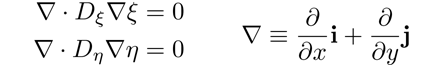The equations take the form of weighted Laplacians, equivalent to diffusion equations with variable coefficients. A more formal discussion is found in this excerpt on crystal growth modeling (Yeckel and Derby, 2005).

Some examples illustrating the effect of these coefficients are shown below. Uniform coefficients give elements that are uniform in size, and variable coefficients give stretched meshes with small elements where the coefficients are small and vice-versa. Stretching can be varied in each coordinate direction independently.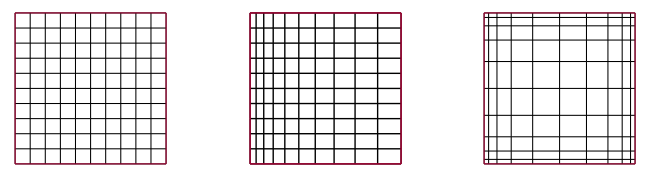These coefficients can be computed from the initial mesh regardless of how it was obtained.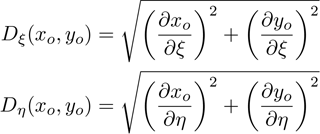The coefficients are also scaled to allow a jump in element density between neighboring regions, which preserves the spacing of the initial mesh, as shown on the left where the EMG mesh matches the initial mesh. If the coefficients are set to unity, the EMG equations will try to equalize the elements in size between the neighboring regions. If the boundary nodes are fixed by Dirichlet conditions, equalization cannot be fully attained and element shapes become distorted near the jump, as shown in the middle. If the nodes are allowed to slide along the boundary, the element size will equalize everywhere, as shown on the right.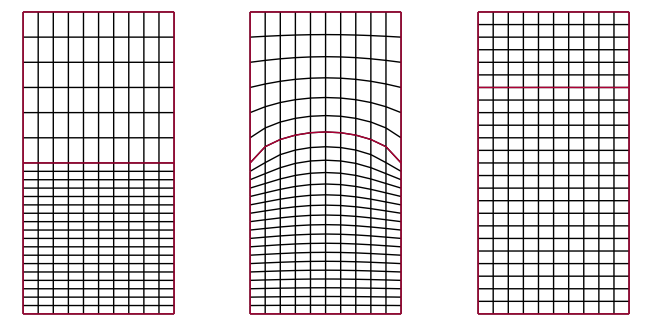If the elements are skewed, transfinite interpolation gives a desirable result (left) whereas EMG with natural boundary conditions over-emphasizes orthogonality (middle). Applying a shearing boundary condition can largely mitigate the problem (right), which is done automatically in Cats2D.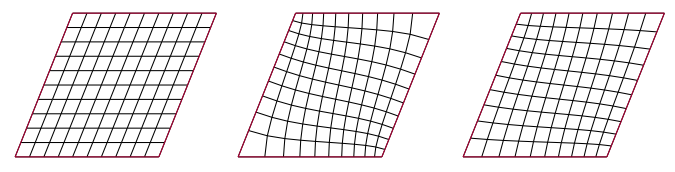This is all pretty simple stuff until we try to patch together several of these quadrilateral mesh regions to form an irregular topology such as the mesh on a circular domain.

Intersection of three grid lines at a point

The devil is in the details, as the saying goes. Shown here are two ways to divide a semicircular domain into quadrilateral regions for the purpose of mesh generation. The red lines show which boundaries in the computational space are merged in the physical space. The first topology shows how the the north sides of regions on the left and right can be merged with the east and west sides of the upper region. The second topology shows how the south sides of regions on the left and right can be merged with east and west sides of the lower region.These merged boundaries don't pose any problems when the position of the nodes there are fixed by Dirichlet conditions. But this cannot be done for situations in which the domain deforms away from its initial circular shape, as will happen to a drop or bubble subjected to shear or body forces, or to an inclusion of liquid metal in a binary alloy. If we cannot rely on the EMG equations to control movement of these boundaries, we must impose ad hoc geometric constraints on them instead, an awkward situation we prefer to avoid.

Below on the left is shown an initial mesh computed by transfinite interpolation for the first topology illustrated above. Shown in the middle is the mesh computed by EMG without imposing any special treatment along the merged boundary. A close up of the intersection of three mesh regions is shown on the right. This mesh is reasonably good, but obtuse angles have formed in the elements at the intersection that suggest failure will occur should the domain deform significantly.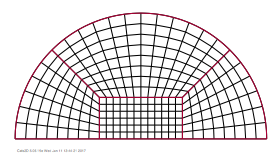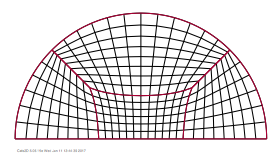In this mesh topology, elements along one side of the merge have their computational coordinates Xi and Eta swapped with respect to the elements on the other side. Ordinarily contributions in the Xi computational direction are added to the X residual equation, and contributions to the Eta computational direction are added to the Y residual equation. Along the merge, however, Xi contributions on one side are added to Eta contributions from the other side for the X residual, and vice-versa for the Y-residual. This tends to maintain a square angle between lines of constant Xi and Eta on opposite sides of the merge.

We can improve the situation for this mesh topology by swapping residual contributions along the merge to pair up Xi and Eta contributions correctly. Below on the left is shown the mesh obtained by EMG with residual swapping. The Xi and Eta coordinates along the merge tend to align rather than square off, which gives a more pleasing result than seen above. Three close ups show the merge corner for three different treatments at this node. On the left is shown the preferred treatment, in which one of the residual contributions along the merge is suppressed. If residuals are swapped at this node without suppressing any contributions, one of the elements at the intersection "folds" making the mapping singular, which is shown in the middle close up. To the right is shown what happens if the swapping is not done at all. The result is acceptable here, but tests show that it tends to fail easily if the element size changes much around the merge corner.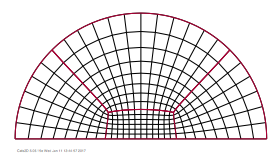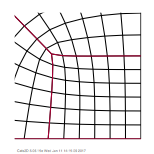We show next the same results repeated for the second topology. Below on the left is shown transfinite interpolation, which behaves identically on either topology. In the middle is shown EMG. The location of the merged boundaries is different in this topology, but the effect of mismatched Xi and Eta residual contributions along it is similar to before.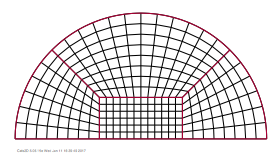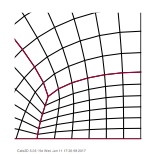Swapping residuals along the merge with the same three corner treatments tested before is shown below. The preferred treatment gives a result very similar to the first topology (left close up), but the alternative treatments (middle and right close ups) behave opposite what was observed before, indicative of the lack of reliability of these treatments.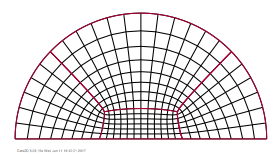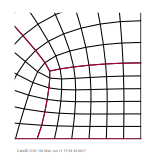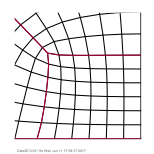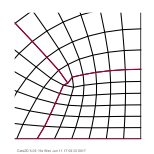Next I introduce a more complicated situation of a circular domain embedded in a larger domain, the mesh for which has another type of irregular point in which five grid lines intersect.

Intersection of five grid lines at a point

Here I look at the situation of a semicircular region embedded in a larger domain, which introduces an irregular point at which five grid lines intersect. The goal of these circle-in-a-square meshes is to solve problems in which the circle is a free boundary, perhaps a gas bubble in a liquid, or a liquid inclusion in a solid, that deforms under physics. We prefer a method that is robust to deformation without requiring a lot of user intervention. The tests performed here impose no internal geometric controls on the mesh region boundaries, allowing the initial circular shape to deform subject to the behavior of the EMG equations.

Results shown below compare the initial mesh computed by transfinite interpolation, on the left, with the mesh computed by EMG without any residual swapping, on the the right: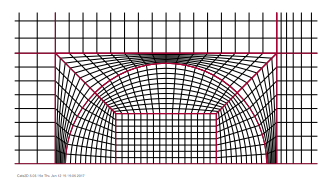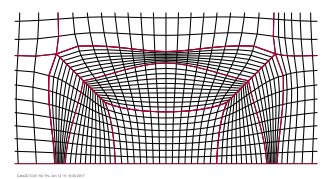The results show the same tendency to "square off" the circle at the merge boundaries observed above for the semicircular domain, except that here the effect extends to the boundary of the circle, which is no longer constrained to retain a circular shape. At the points where five grid lines intersect, the equations give a reasonable if ugly result. The points at which three grid lines intersect present the same difficulties as before.

If residuals are swapped along the merge boundaries, but no swapping is done at the merge corners, the result shown below is acceptable, but just as before this approach is prone to failure if the mesh density changes much between regions: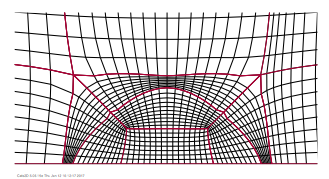The initial circular shape of the boundary is approximately maintained, which is a big improvement over the previous result, but a slight corner has bulged outwards where the circle intersects the merge boundary. This corner goes away if the residuals at the merge corners are swapped without suppressing any contributions, as seen below, but now one of the elements "folds" making the mapping singular: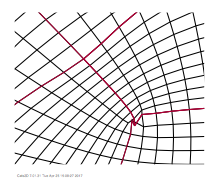This is the same problem observed in the case of three grid lines intersecting, and the preferred treatment identified there works here as well, which is to suppress one of the residual contributions at the merge corners. Doing this gives a good result that is robust to large changes in element size between regions: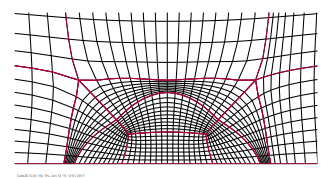Throughout these tests the points at which five grid lines intersect behave less problematically than the points at which three grid lines intersect. All three treatments give an acceptable result at these points, although the most pleasing result is produced by the preferred treatment.

Here is a test of another domain shape featuring irregular points of this type, showing an initial mesh by transfinite interpolation (below left), a mesh computed without residual swapping at the merge boundaries (below middle), and a mesh computed using residual swapping along the merge boundaries (below right):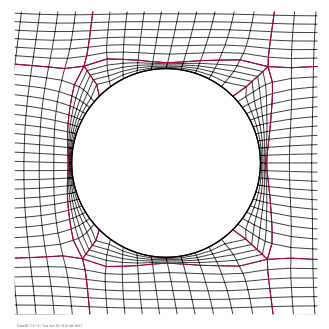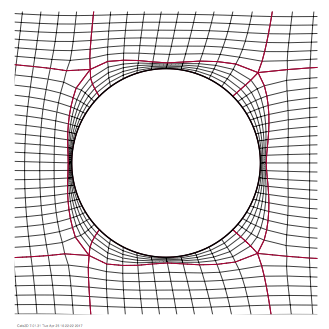Again, a better mesh is obtained when residuals are swapped at the merge boundaries. I think I have managed to cook up a simple scheme to handle these awkward mesh topologies without needing to impose ad hoc geometric constraints on the internal mesh boundaries. The results aren't particularly pretty but are usable. The next step is to test these schemes on a physical problem with an interface of some sort.

Putting myself through all this makes me question the utility of elliptic mesh generation. EMG is something we got locked into about 25 years ago when we started this project, and we never looked back. We have tended towards studying problems of fairly simple geometry, and we have accepted the occasional burden of manually laying out the mesh regions to suit the domain at hand. But EMG forces us to use structured grids, which greatly constrains the manner and degree to which the mesh can deform from its initial shape without becoming singular. If I could roll back the clock knowing what I do now, I would favor an unstructured method of some type, but I find the idea of retrofitting today's Cats2D too daunting to consider.